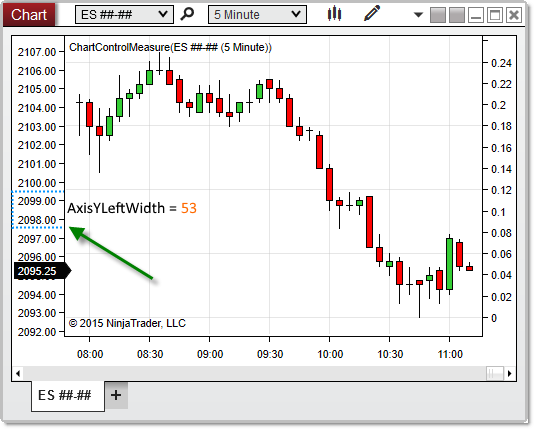﻿ NinjaScript > Language Reference > Common > Charts > ChartControl > AxisYLeftWidth

AxisYLeftWidth

 Navigation:  NinjaScript > Language Reference > Common > Charts > ChartControl > AxisYLeftWidthDefinition

Measures the distance (in pixels) between the y-axis and the left edge of a chart.

Property Value

A double representing the number of pixels separating the y-axis and the left edge of the chart.

Syntax

<ChartControl>.AxisYLeftWidthprotected override void OnRender(ChartControl chartControl, ChartScale chartScale)
{
// Print the number of pixels between the y-axis and the left edge of the chart
double leftWidth = chartControl.AxisYLeftWidth;
Print(leftWidth);
}

Based on the image below, AxisYLeftWidth reveals that the space between the y-axis and the left edge of the chart is 53 pixels on this chart.Note: When there are no left-justified data series on a chart, AxisYLeftWidth will return 0, as there will be no space between the y-axis and the left margin.